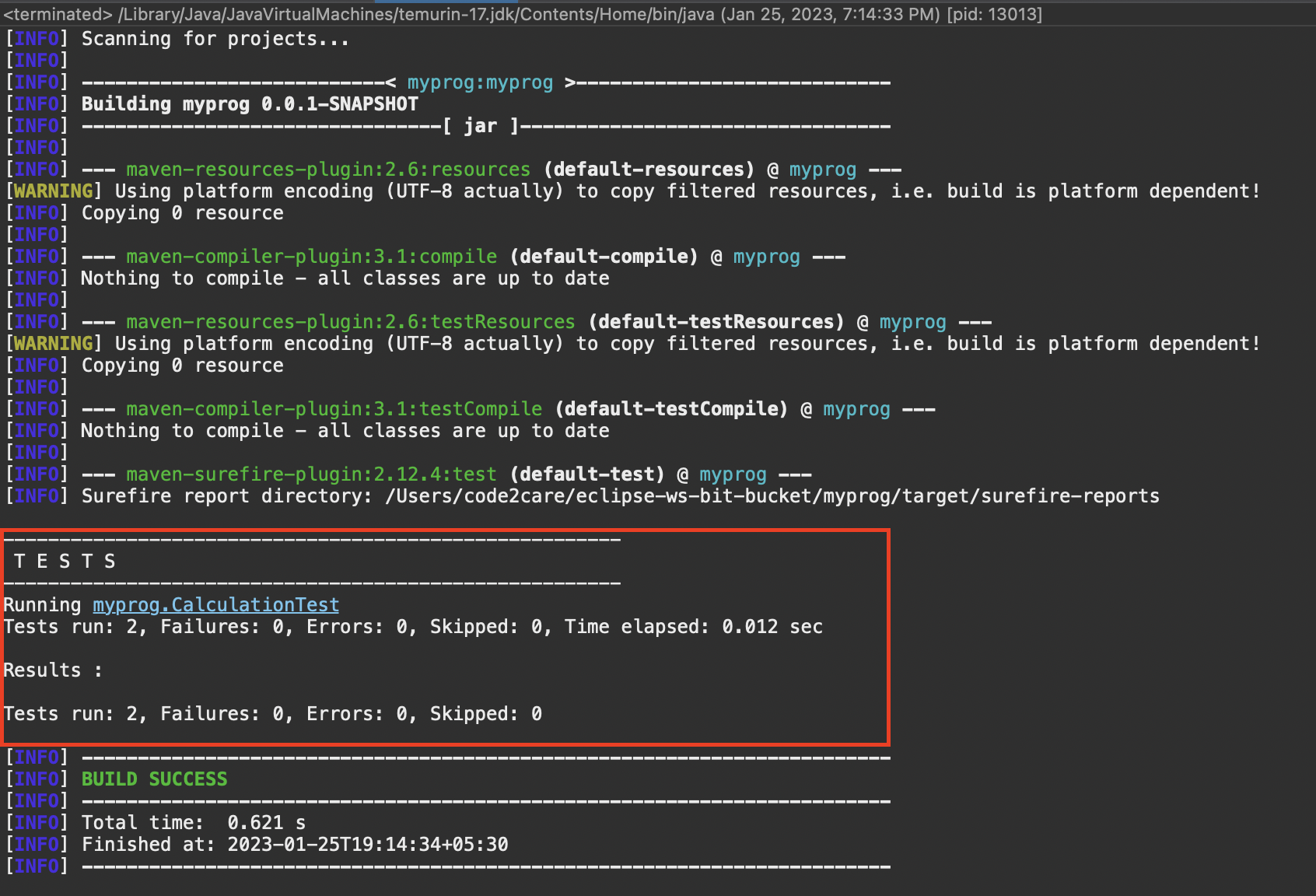# How to test Exceptions using Java JUnit

Let us take a look at how to test exceptions using Junit.

Calculation.java
``````package org.code2care;

public class Calculation {

public int divide(int no1, int no2) {
return no1 / no2;
}

public void numberToString(int no) {
System.out.println("The no. entered is: " + Integer.toString(no));

}
}
``````
CalculationClient.java
``````package org.code2care;

public class CalculationClient {

public static void main(String[] args) {

Calculation cal = new Calculation();

Integer no1 = 20;
Integer no2 = 10;

System.out.println(cal.divide(no1, no2));
cal.numberToString(no2);

}

}``````

We have two methods division that can throw ArithmeticException and numberToString that can throw a NullPointerException

Let's write test cases in Java JUnit to test these exceptions.

CalculationTest.java
``````package myprog;

import org.code2care.Calculation;
import org.junit.Test;

/**
*
* JUnit test cases for
* testing exceptions
*
* @author code2care
*
*/
public class CalculationTest {

@Test(expected = ArithmeticException.class)
public void divideTwoNumbersArithmeticExceptionTest() {
Calculation calculation = new Calculation();
int no1 = 20;
int no2 = 0;
calculation.divide(no1, no2);
}

@Test(expected = NullPointerException.class)
public void noToStringNullPointerExceptionText() {
Calculation calculation = new Calculation();
Integer no1 = null;
calculation.numberToString(no1);
}

}
``````-

Have Questions? Post them here!